# Hearts

5 cards are chosen from a standard deck of 52 playing cards (13 hearts) with replacement. What is the probability of choosing 5 hearts in a row?

Result

p =  0.1 %

#### Solution:Leave us a comment of example and its solution (i.e. if it is still somewhat unclear...):Be the first to comment!#### To solve this example are needed these knowledge from mathematics:

Would you like to compute count of combinations?

## Next similar examples:

1. CardsThe player gets 8 cards of 32. What is the probability that it gets a) all 4 aces b) at least 1 ace
2. CardsSuppose that are three cards in the hats. One is red on both sides, one of which is black on both sides, and a third one side red and the second black. We are pulled out of a hat randomly one card and we see that one side of it is red. What is the probabi
3. Class - boys and girls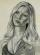In the class are 60% boys and 40% girls. Long hair has 10% boys and 80% girls. a) What is the probability that a randomly chosen person has long hair? b) The selected person has long hair. What is the probability that it is a girl?
4. BallsFrom the urn in which are 7 white balls and 17 red, gradually drag 3-times without replacement. What is the probability that pulls balls are in order: red red red?
5. Theorem proveWe want to prove the sentence: If the natural number n is divisible by six, then n is divisible by three. From what assumption we started?
6. Green - Red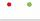We have 5 bags. Each consist one green and 2 red balls. From each we pull just one ball. What is the probability that we doesn't pull any green ball?
7. Lottery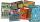Fernando has two lottery tickets each from other lottery. In the first is 973 000 lottery tickets from them wins 687 000, the second has 1425 000 lottery tickets from them wins 1425 000 tickets. What is the probability that at least one Fernando's ticket w
8. Normal distribution GPA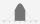The average GPA is 2.78 with a standard deviation of 4.5. What are students in the bottom the 20% having what GPA?
9. A bookA book contains 524 pages. If it is known that a person will select any one page between the pages numbered 125 and 384, find the probability of choosing the page numbered 252 or 253.
10. A studentA student is to answer 8 out of 10 questions on the exam. a) find the number n of ways the student can choose 8 out of 10 questions b) find n if the student must answer the first three questions c) How many if he must answer at least 4 of the first 5 queOn the menu are 12 kinds of meal. How many ways can we choose four different meals into the daily menu?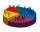We have n identical balls (numbered 1-n) is selected without replacement. Determine 1) The probability that at least one tensile strength number coincides with the number of balls? 2) Determine the mean and variance of the number of balls, which coincides.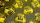What are the chances that the lottery, in which the numbers are drawn 5 of 50 you win the first prize?There are 9 girls and 11 boys in the class today. What is the probability that Suzan will go to the board today?How many five-digit PIN - code can we create using the even numbers?Division has 18 members: 10 girls and 6 boys, 2 leaders. How many different patrols can be created, if one patrol is 2 boys, 3 girls and 1 leader?In the container are 45 white and 15 balls. We randomly select 5 balls. What is the probability that it will be a maximum one green?